## 9.4 Polyatomic Systems with Multiple Bonds

### Learning Objective

1. To explain resonance structures using molecular orbitals.

So far in our molecular orbital descriptions we have not dealt with polyatomic systems with multiple bonds. To do so, we can use an approach in which we describe σ bonding using localized electron-pair bonds formed by hybrid atomic orbitals, and π bonding using molecular orbitals formed by unhybridized np atomic orbitals.

## Multiple Bonds

We begin our discussion by considering the bonding in ethylene (C2H4). Experimentally, we know that the H–C–H and H–C–C angles in ethylene are approximately 120°. This angle suggests that the carbon atoms are sp2 hybridized, which means that a singly occupied sp2 orbital on one carbon overlaps with a singly occupied s orbital on each H and a singly occupied sp2 lobe on the other C. Thus each carbon forms a set of three σ bonds: two C–H (sp2 + s) and one C–C (sp2 + sp2) (part (a) in Figure 9.32 "Bonding in Ethylene"). The sp2 hybridization can be represented as follows: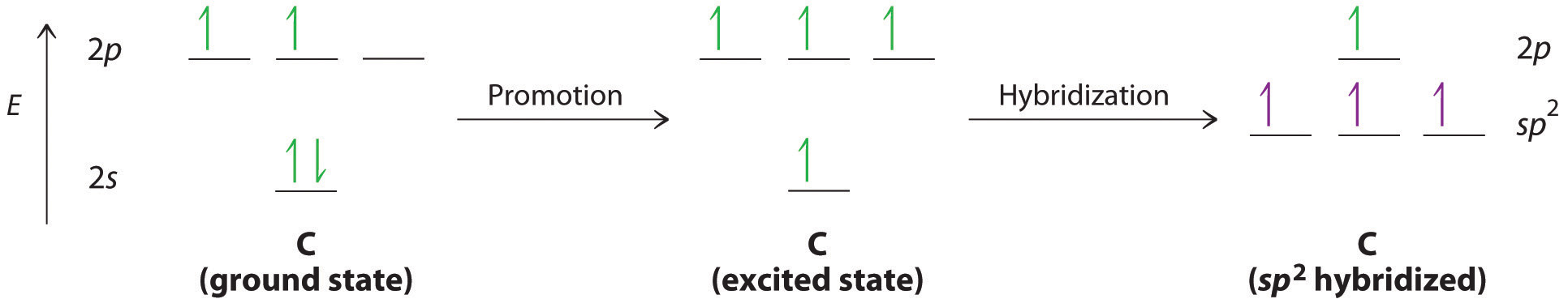Figure 9.32 Bonding in Ethylene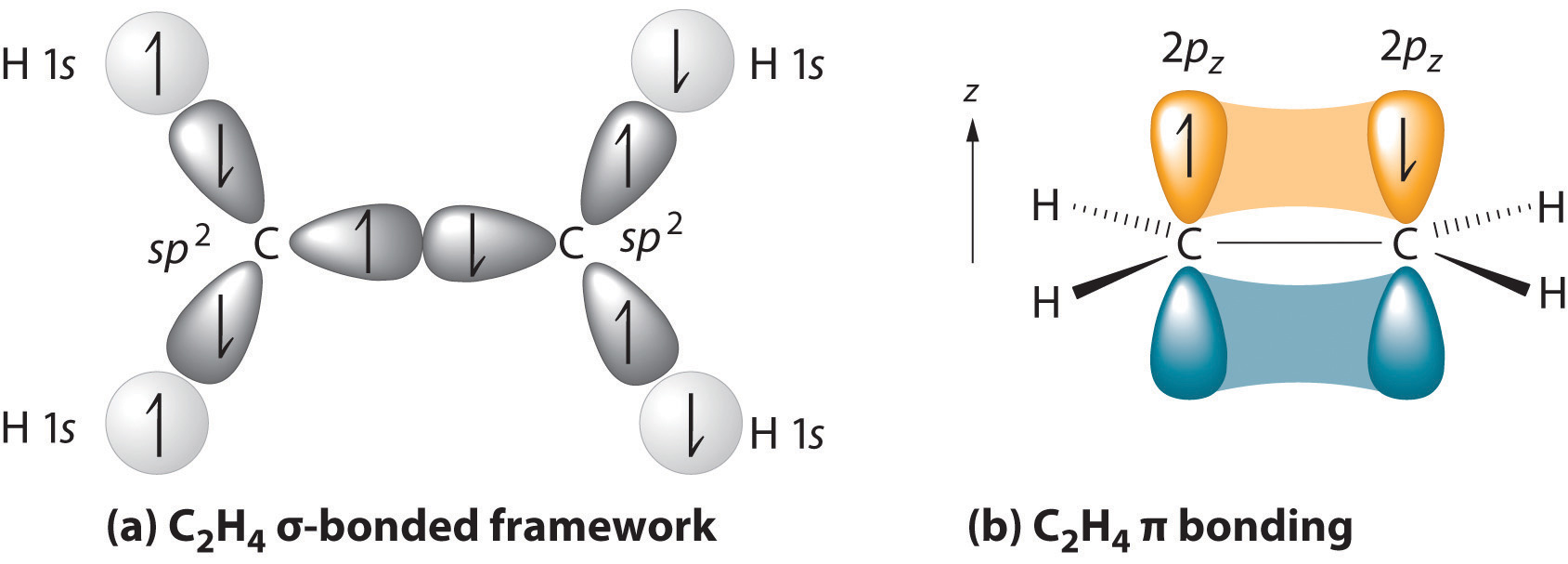(a) The σ-bonded framework is formed by the overlap of two sets of singly occupied carbon sp2 hybrid orbitals and four singly occupied hydrogen 1s orbitals to form electron-pair bonds. This uses 10 of the 12 valence electrons to form a total of five σ bonds (four C–H bonds and one C–C bond). (b) One singly occupied unhybridized 2pz orbital remains on each carbon atom to form a carbon–carbon π bond. (Note: by convention, in planar molecules the axis perpendicular to the molecular plane is the z-axis.)

After hybridization, each carbon still has one unhybridized 2pz orbital that is perpendicular to the hybridized lobes and contains a single electron (part (b) in Figure 9.32 "Bonding in Ethylene"). The two singly occupied 2pz orbitals can overlap to form a π bonding orbital and a π* antibonding orbital, which produces the energy-level diagram shown in Figure 9.33 "Molecular Orbital Energy-Level Diagram for π Bonding in Ethylene". With the formation of a π bonding orbital, electron density increases in the plane between the carbon nuclei. The π* orbital lies outside the internuclear region and has a nodal plane perpendicular to the internuclear axis. Because each 2pz orbital has a single electron, there are only two electrons, enough to fill only the bonding (π) level, leaving the π* orbital empty. Consequently, the C–C bond in ethylene consists of a σ bond and a π bond, which together give a C=C double bond. Our model is supported by the facts that the measured carbon–carbon bond is shorter than that in ethane (133.9 pm versus 153.5 pm) and the bond is stronger (728 kJ/mol versus 376 kJ/mol in ethane). The two CH2 fragments are coplanar, which maximizes the overlap of the two singly occupied 2pz orbitals.

Figure 9.33 Molecular Orbital Energy-Level Diagram for π Bonding in Ethylene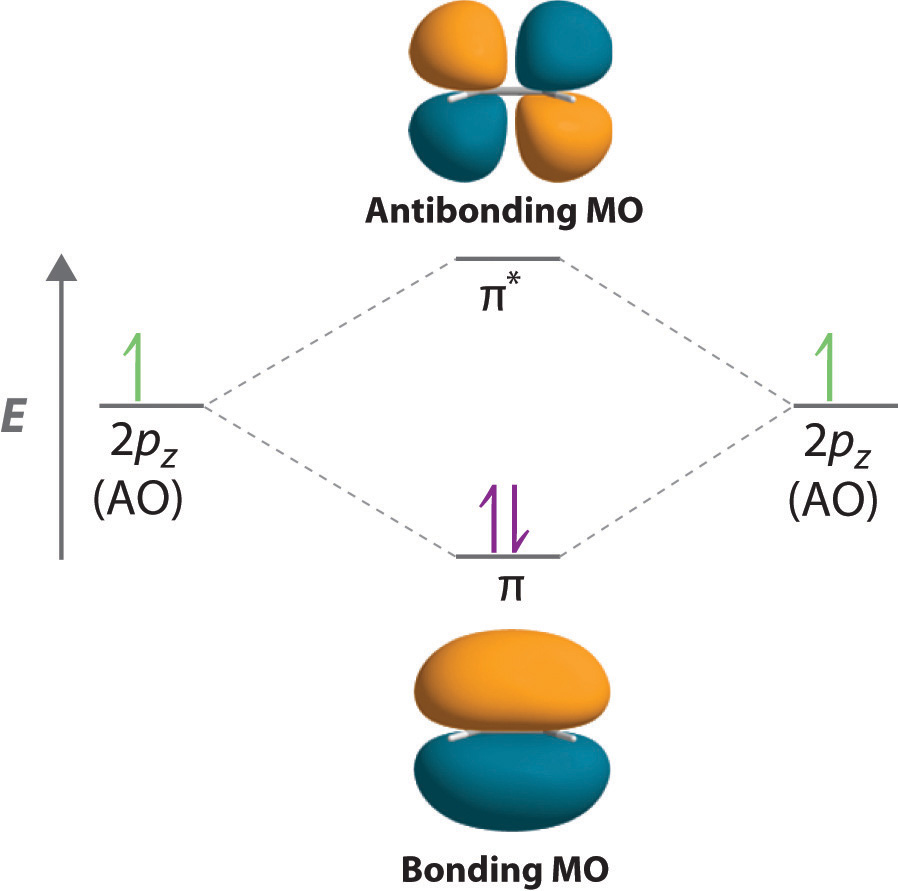As in the diatomic molecules discussed previously, the singly occupied 2pz orbitals in ethylene can overlap to form a bonding/antibonding pair of π molecular orbitals. The two electrons remaining are enough to fill only the bonding π orbital. With one σ bond plus one π bond, the carbon–carbon bond order in ethylene is 2.

Triple bonds, as in acetylene (C2H2), can also be explained using a combination of hybrid atomic orbitals and molecular orbitals. The four atoms of acetylene are collinear, which suggests that each carbon is sp hybridized. If one sp lobe on each carbon atom is used to form a C–C σ bond and one is used to form the C–H σ bond, then each carbon will still have two unhybridized 2p orbitals (a 2px,y pair), each with one electron (part (a) in Figure 9.34 "Bonding in Acetylene").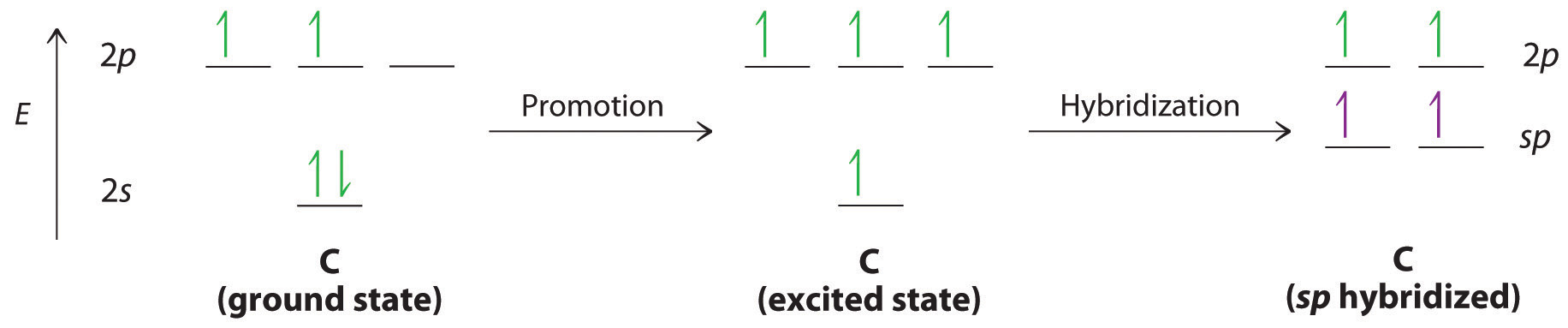The two 2p orbitals on each carbon can align with the corresponding 2p orbitals on the adjacent carbon to simultaneously form a pair of π bonds (part (b) in Figure 9.34 "Bonding in Acetylene"). Because each of the unhybridized 2p orbitals has a single electron, four electrons are available for π bonding, which is enough to occupy only the bonding molecular orbitals. Acetylene must therefore have a carbon–carbon triple bond, which consists of a C–C σ bond and two mutually perpendicular π bonds. Acetylene does in fact have a shorter carbon–carbon bond (120.3 pm) and a higher bond energy (965 kJ/mol) than ethane and ethylene, as we would expect for a triple bond.

Figure 9.34 Bonding in Acetylene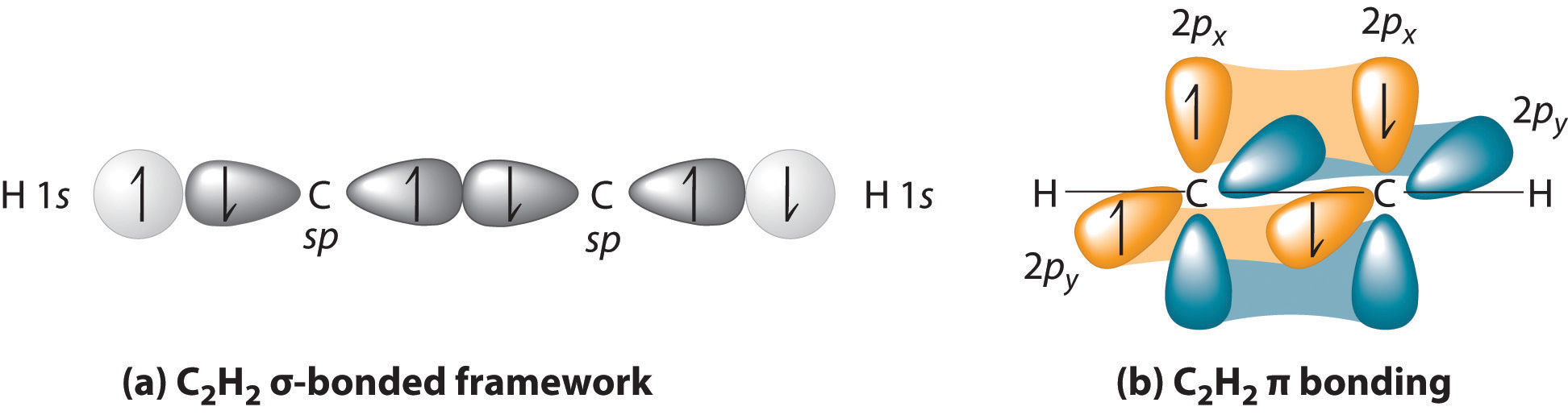(a) In the formation of the σ-bonded framework, two sets of singly occupied carbon sp hybrid orbitals and two singly occupied hydrogen 1s orbitals overlap. (b) In the formation of two carbon–carbon π bonds in acetylene, two singly occupied unhybridized 2px,y orbitals on each carbon atom overlap. With one σ bond plus two π bonds, the carbon–carbon bond order in acetylene is 3.

### Note the Pattern

In complex molecules, hybrid orbitals and valence bond theory can be used to describe σ bonding, and unhybridized π orbitals and molecular orbital theory can be used to describe π bonding.

### Example 12

Describe the bonding in HCN using a combination of hybrid atomic orbitals and molecular orbitals. The HCN molecule is linear.

Given: chemical compound and molecular geometry

Asked for: bonding description using hybrid atomic orbitals and molecular orbitals

Strategy:

A From the geometry given, predict the hybridization in HCN. Use the hybrid orbitals to form the σ-bonded framework of the molecule and determine the number of valence electrons that are used for σ bonding.

B Determine the number of remaining valence electrons. Use any remaining unhybridized p orbitals to form π and π* orbitals.

C Fill the orbitals with the remaining electrons in order of increasing energy. Describe the bonding in HCN.

Solution:

A Because HCN is a linear molecule, it is likely that the bonding can be described in terms of sp hybridization at carbon. Because the nitrogen atom can also be described as sp hybridized, we can use one sp hybrid on each atom to form a C–N σ bond. This leaves one sp hybrid on each atom to either bond to hydrogen (C) or hold a lone pair of electrons (N). Of 10 valence electrons (5 from N, 4 from C, and 1 from H), 4 are used for σ bonding:B We are now left with 2 electrons on N (5 valence electrons minus 1 bonding electron minus 2 electrons in the lone pair) and 2 electrons on C (4 valence electrons minus 2 bonding electrons). We have two unhybridized 2p atomic orbitals left on carbon and two on nitrogen, each occupied by a single electron. These four 2p atomic orbitals can be combined to give four molecular orbitals: two π (bonding) orbitals and two π* (antibonding) orbitals. C With 4 electrons available, only the π orbitals are filled. The overall result is a triple bond (1 σ and 2 π) between C and N.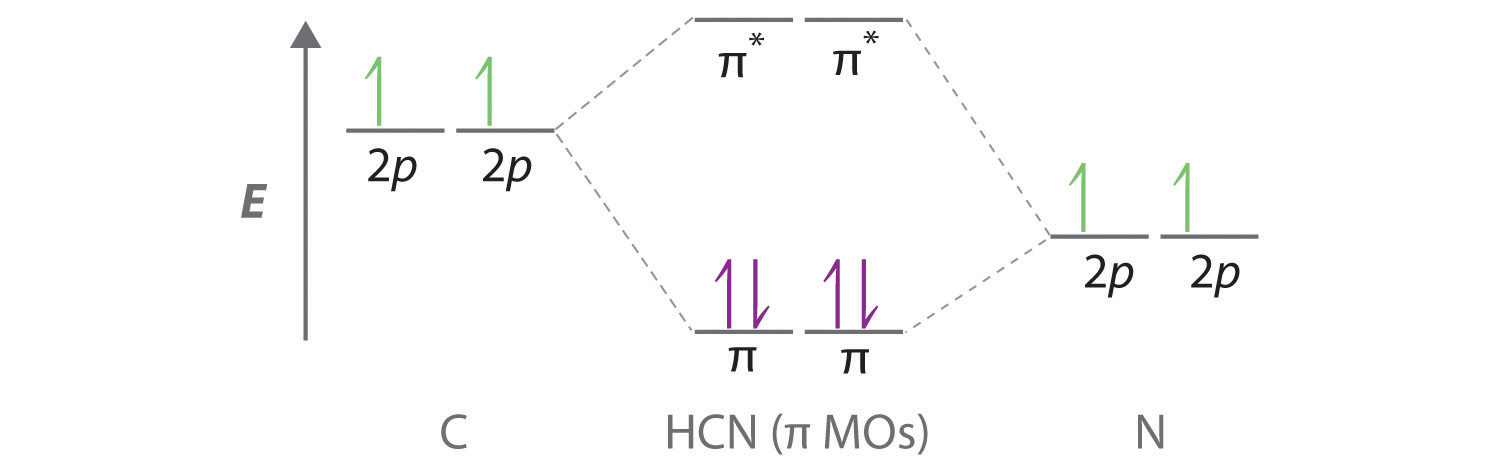Exercise

Describe the bonding in formaldehyde (H2C=O), a trigonal planar molecule, using a combination of hybrid atomic orbitals and molecular orbitals.

σ-bonding framework: Carbon and oxygen are sp2 hybridized. Two sp2 hybrid orbitals on oxygen have lone pairs, two sp2 hybrid orbitals on carbon form C–H bonds, and one sp2 hybrid orbital on C and O forms a C–O σ bond.

π bonding: Unhybridized, singly occupied 2p atomic orbitals on carbon and oxygen interact to form π (bonding) and π* (antibonding) molecular orbitals. With two electrons, only the π (bonding) orbital is occupied.

## Molecular Orbitals and Resonance Structures

In Chapter 8 "Ionic versus Covalent Bonding", we used resonance structures to describe the bonding in molecules such as ozone (O3) and the nitrite ion (NO2). We showed that ozone can be represented by either of these Lewis electron structures:Although the VSEPR model correctly predicts that both species are bent, it gives no information about their bond orders.

Figure 9.35 Bonding in Ozone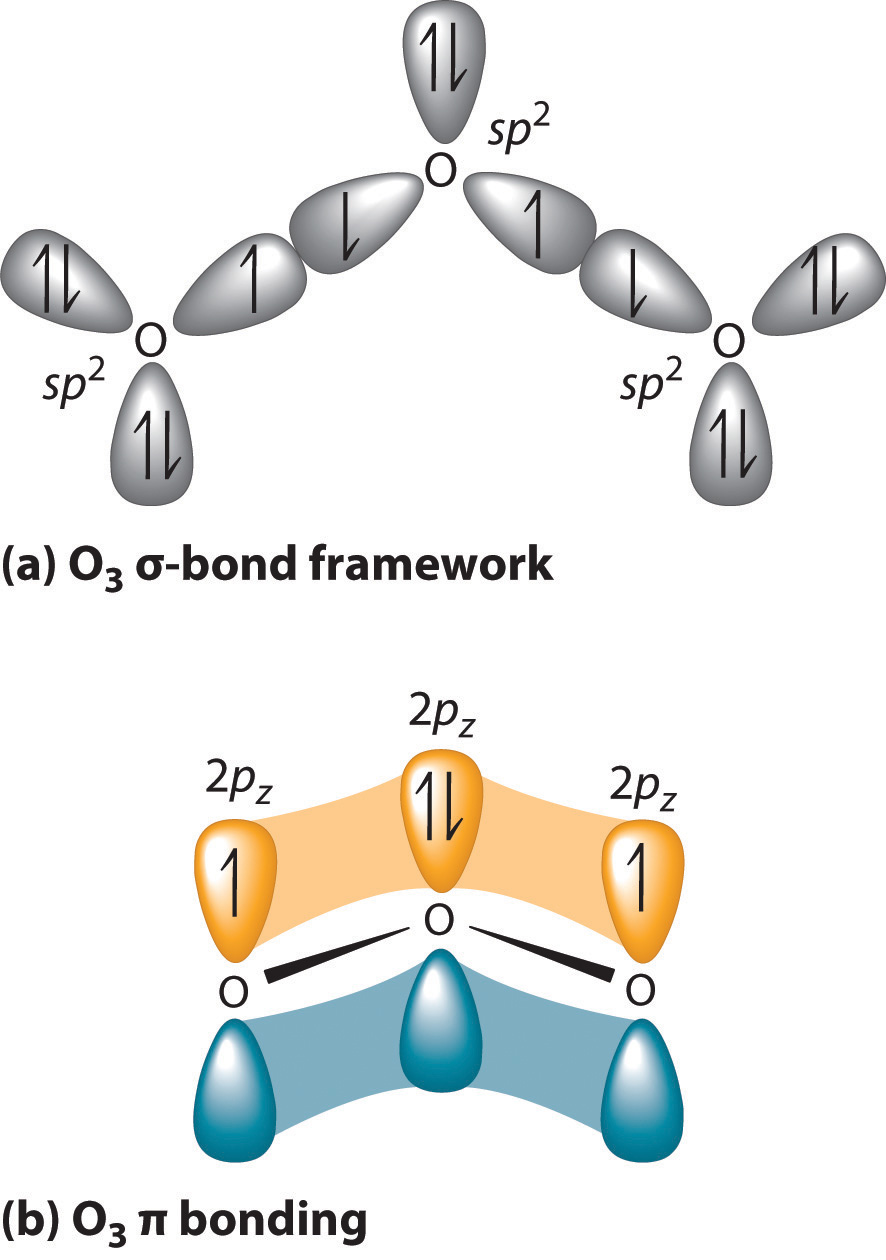(a) In the formation of the σ-bonded framework, three sets of oxygen sp2 hybrid orbitals overlap to give two O–O σ bonds and five lone pairs, two on each terminal O and one on the central O. The σ bonds and lone pairs account for 14 of the 18 valence electrons of O3. (b) One unhybridized 2pz orbital remains on each oxygen atom that is available for π bonding. The unhybridized 2pz orbital on each terminal O atom has a single electron, whereas the unhybridized 2pz orbital on the central O atom has 2 electrons.

Experimental evidence indicates that ozone has a bond angle of 117.5°. Because this angle is close to 120°, it is likely that the central oxygen atom in ozone is trigonal planar and sp2 hybridized. If we assume that the terminal oxygen atoms are also sp2 hybridized, then we obtain the σ-bonded framework shown in Figure 9.35 "Bonding in Ozone". Two of the three sp2 lobes on the central O are used to form O–O sigma bonds, and the third has a lone pair of electrons. Each terminal oxygen atom has two lone pairs of electrons that are also in sp2 lobes. In addition, each oxygen atom has one unhybridized 2p orbital perpendicular to the molecular plane. The σ bonds and lone pairs account for a total of 14 electrons (five lone pairs and two σ bonds, each containing 2 electrons). Each oxygen atom in ozone has 6 valence electrons, so O3 has a total of 18 valence electrons. Subtracting 14 electrons from the total gives us 4 electrons that must occupy the three unhybridized 2p orbitals.

With a molecular orbital approach to describe the π bonding, three 2p atomic orbitals give us three molecular orbitals, as shown in Figure 9.36 "π Bonding in Ozone". One of the molecular orbitals is a π bonding molecular orbital, which is shown as a banana-shaped region of electron density above and below the molecular plane. This region has no nodes perpendicular to the O3 plane. The molecular orbital with the highest energy has two nodes that bisect the O–O σ bonds; it is a π* antibonding orbital. The third molecular orbital contains a single node that is perpendicular to the O3 plane and passes through the central O atom; it is a nonbonding molecular orbital. Because electrons in nonbonding orbitals are neither bonding nor antibonding, they are ignored in calculating bond orders.

Figure 9.36 π Bonding in Ozone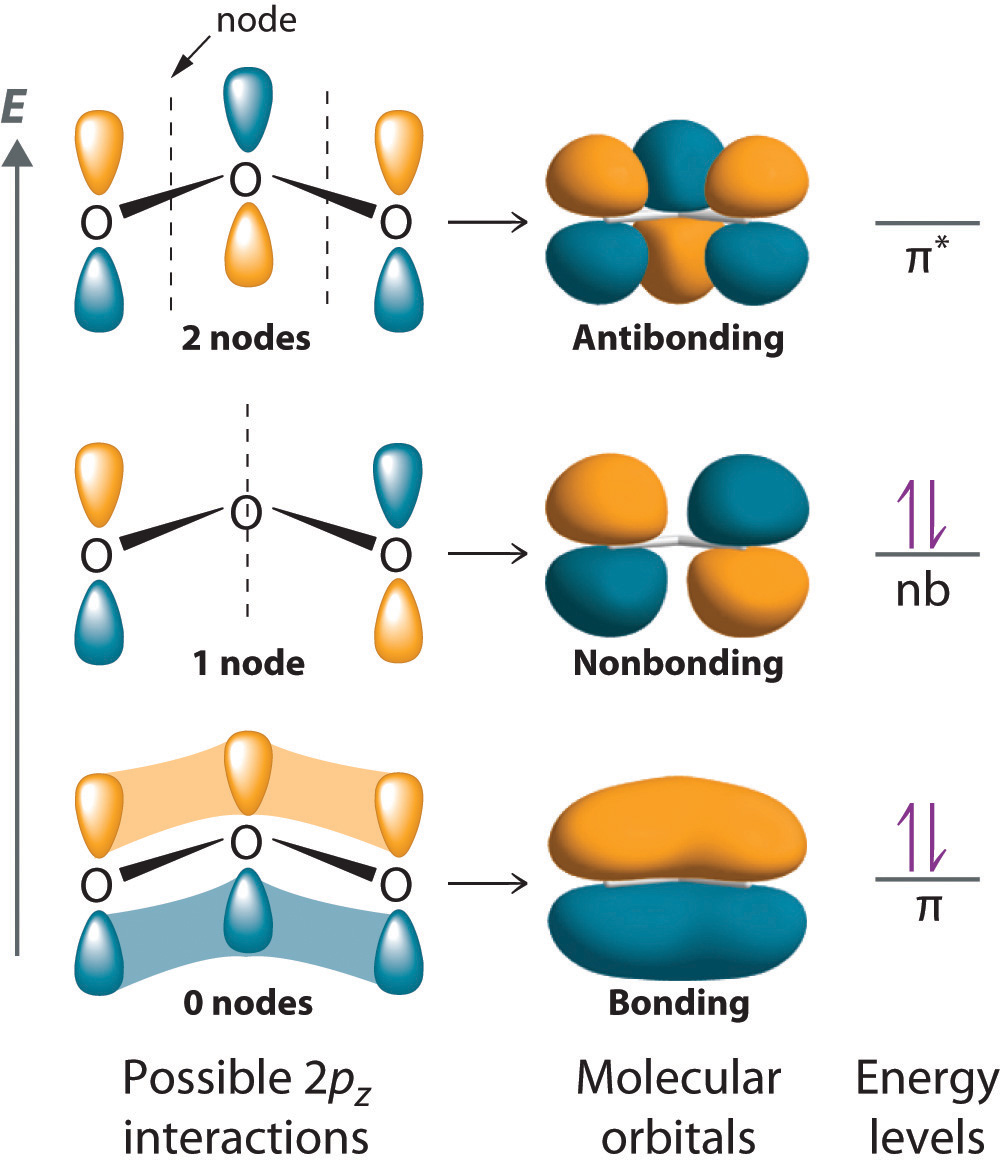The three unhybridized 2pz atomic orbitals interact with one another to form three molecular orbitals: one π bonding orbital at lower energy, one π* antibonding orbital at higher energy, and a nonbonding orbital in between. Placing four electrons in this diagram fills the bonding and nonbonding orbitals. With one filled π bonding orbital holding three atoms together, the net π bond order is $12$ per O–O bond. The combined σ/π bond order is thus $112$ for each O–O bond.

We can now place the remaining four electrons in the three energy levels shown in Figure 9.36 "π Bonding in Ozone", thereby filling the π bonding and the nonbonding levels. The result is a single π bond holding three oxygen atoms together, or $12π$ bond per O–O. We therefore predict the overall O–O bond order to be $112$ ($12π$ bond plus 1 σ bond), just as predicted using resonance structures. The molecular orbital approach, however, shows that the π nonbonding orbital is localized on the terminal O atoms, which suggests that they are more electron rich than the central O atom. The reactivity of ozone is consistent with the predicted charge localization.

### Note the Pattern

Resonance structures are a crude way of describing molecular orbitals that extend over more than two atoms.

### Example 13

Describe the bonding in the nitrite ion in terms of a combination of hybrid atomic orbitals and molecular orbitals. Lewis dot structures and the VSEPR model predict that the NO2 ion is bent.

Given: chemical species and molecular geometry

Asked for: bonding description using hybrid atomic orbitals and molecular orbitals

Strategy:

A Calculate the number of valence electrons in NO2. From the structure, predict the type of atomic orbital hybridization in the ion.

B Predict the number and type of molecular orbitals that form during bonding. Use valence electrons to fill these orbitals and then calculate the number of electrons that remain.

C If there are unhybridized orbitals, place the remaining electrons in these orbitals in order of increasing energy. Calculate the bond order and describe the bonding.

Solution:

A The lone pair of electrons on nitrogen and a bent structure suggest that the bonding in NO2 is similar to the bonding in ozone. This conclusion is supported by the fact that nitrite also contains 18 valence electrons (5 from N and 6 from each O, plus 1 for the −1 charge). The bent structure implies that the nitrogen is sp2 hybridized.

B If we assume that the oxygen atoms are sp2 hybridized as well, then we can use two sp2 hybrid orbitals on each oxygen and one sp2 hybrid orbital on nitrogen to accommodate the five lone pairs of electrons. Two sp2 hybrid orbitals on nitrogen form σ bonds with the remaining sp2 hybrid orbital on each oxygen. The σ bonds and lone pairs account for 14 electrons. We are left with three unhybridized 2p orbitals, one on each atom, perpendicular to the plane of the molecule, and 4 electrons. Just as with ozone, these three 2p orbitals interact to form bonding, nonbonding, and antibonding π molecular orbitals. The bonding molecular orbital is spread over the nitrogen and both oxygen atoms.

C Placing 4 electrons in the energy-level diagram fills both the bonding and nonbonding molecular orbitals and gives a π bond order of 1/2 per N–O bond. The overall N–O bond order is $112,$ consistent with a resonance structure.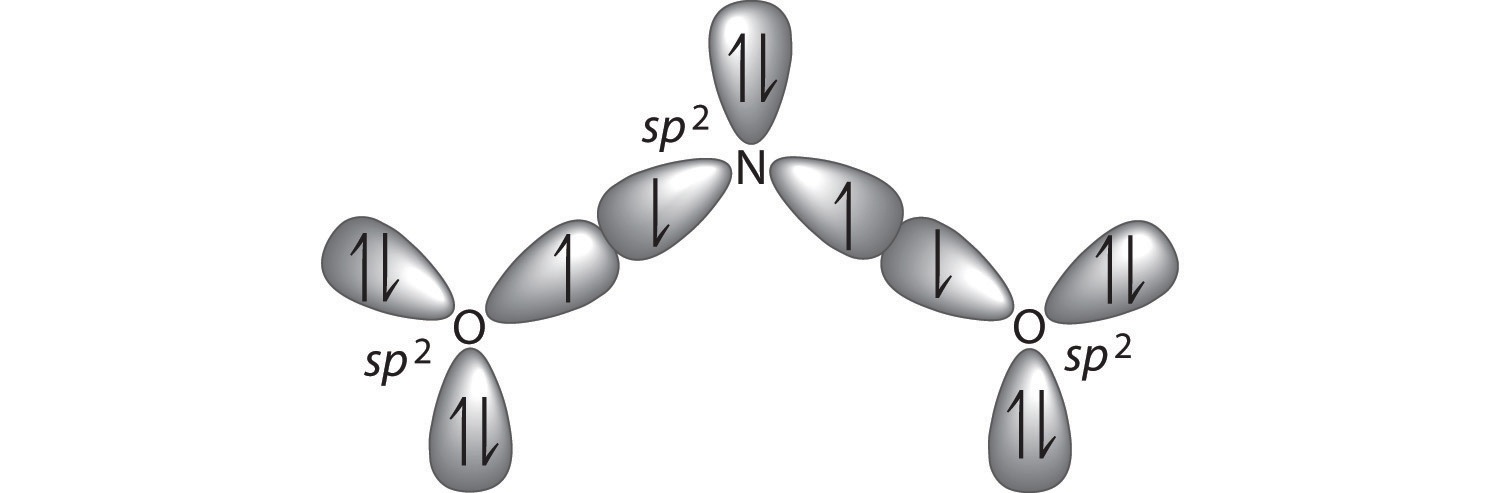Exercise

Describe the bonding in the formate ion (HCO2), in terms of a combination of hybrid atomic orbitals and molecular orbitals.

Answer: Like nitrite, formate is a planar polyatomic ion with 18 valence electrons. The σ bonding framework can be described in terms of sp2 hybridized carbon and oxygen, which account for 14 electrons. The three unhybridized 2p orbitals (on C and both O atoms) form three π molecular orbitals, and the remaining 4 electrons occupy both the bonding and nonbonding π molecular orbitals. The overall C–O bond order is therefore $112.$

## The Chemistry of Vision

Hydrocarbons in which two or more carbon–carbon double bonds are directly linked by carbon–carbon single bonds are generally more stable than expected because of resonance. Because the double bonds are close enough to interact electronically with one another, the π electrons are shared over all the carbon atoms, as illustrated for 1,3-butadiene in Figure 9.37 "π Bonding in 1,3-Butadiene". As the number of interacting atomic orbitals increases, the number of molecular orbitals increases, the energy spacing between molecular orbitals decreases, and the systems become more stable (Figure 9.38 "Molecular Orbital Energy-Level Diagrams for a Chain of "). Thus as a chain of alternating double and single bonds becomes longer, the energy required to excite an electron from the highest-energy occupied (bonding) orbital to the lowest-energy unoccupied (antibonding) orbital decreases. If the chain is long enough, the amount of energy required to excite an electron corresponds to the energy of visible light. For example, vitamin A is yellow because its chain of five alternating double bonds is able to absorb violet light. Many of the colors we associate with dyes result from this same phenomenon; most dyes are organic compounds with alternating double bonds.

Figure 9.37 π Bonding in 1,3-Butadiene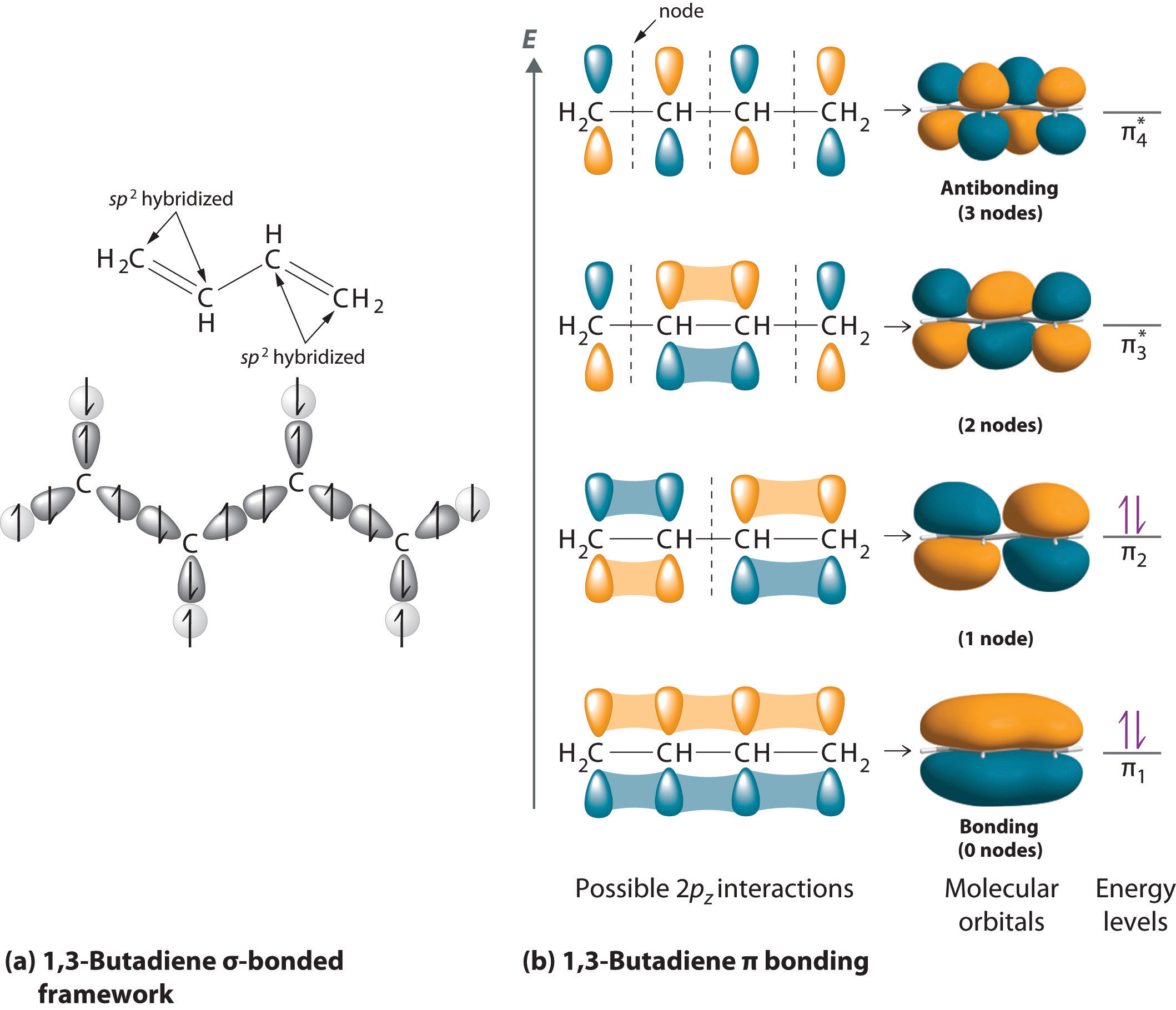(a) If each carbon atom is assumed to be sp2 hybridized, we can construct a σ-bonded framework that accounts for the C–H and C–C single bonds, leaving four singly occupied 2pz orbitals, one on each carbon atom. (b) As in ozone, these orbitals can interact, in this case to form four molecular orbitals. The molecular orbital at lowest energy is a bonding orbital with 0 nodes, the one at highest energy is antibonding with 3 nodes, and the two in the middle have 1 node and 2 nodes and are somewhere between bonding or antibonding and nonbonding, respectively. The energy of the molecular orbital increases with the number of nodes. With four electrons, only the two bonding orbitals are filled, consistent with the presence of two π bonds.

Figure 9.38 Molecular Orbital Energy-Level Diagrams for a Chain of n Like Orbitals That Interact (n ≤ 5)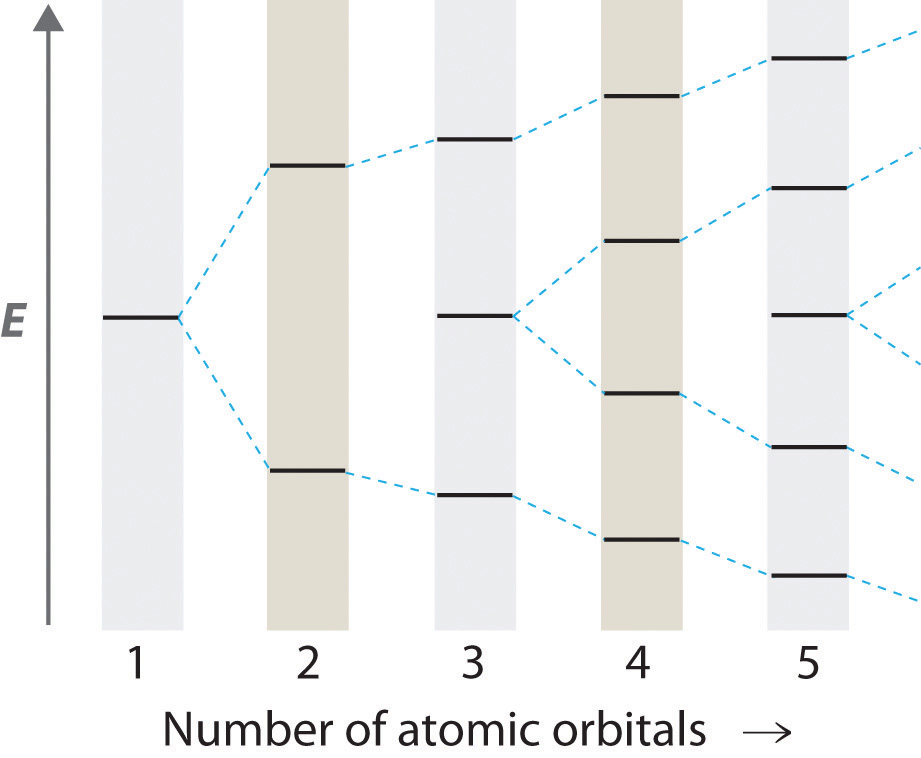As the number of atomic orbitals increases, the difference in energy between the resulting molecular orbital energy levels decreases, which allows light of lower energy to be absorbed. As a result, organic compounds with long chains of carbon atoms and alternating single and double bonds tend to become more deeply colored as the number of double bonds increases.

### Note the Pattern

As the number of interacting atomic orbitals increases, the energy separation between the resulting molecular orbitals steadily decreases.

A derivative of vitamin A called retinal is used by the human eye to detect light and has a structure with alternating C=C double bonds. When visible light strikes retinal, the energy separation between the molecular orbitals is sufficiently close that the energy absorbed corresponds to the energy required to change one double bond in the molecule from cis, where like groups are on the same side of the double bond, to trans, where they are on opposite sides, initiating a process that causes a signal to be sent to the brain. If this mechanism is defective, we lose our vision in dim light. Once again, a molecular orbital approach to bonding explains a process that cannot be explained using any of the other approaches we have described.

### Summary

To describe the bonding in more complex molecules with multiple bonds, we can use an approach that uses hybrid atomic orbitals to describe the σ bonding and molecular orbitals to describe the π bonding. In this approach, unhybridized np orbitals on atoms bonded to one another are allowed to interact to produce bonding, antibonding, or nonbonding combinations. For π bonds between two atoms (as in ethylene or acetylene), the resulting molecular orbitals are virtually identical to the π molecular orbitals in diatomic molecules such as O2 and N2. Applying the same approach to π bonding between three or four atoms requires combining three or four unhybridized np orbitals on adjacent atoms to generate π bonding, antibonding, and nonbonding molecular orbitals extending over all of the atoms. Filling the resulting energy-level diagram with the appropriate number of electrons explains the bonding in molecules or ions that previously required the use of resonance structures in the Lewis electron-pair approach.

### Key Takeaway

• Polyatomic systems with multiple bonds can be described using hybrid atomic orbitals for σ bonding and molecular orbitals to describe π bonding.

### Conceptual Problems

1. What information is obtained by using the molecular orbital approach to bonding in O3 that is not obtained using the VSEPR model? Can this information be obtained using a Lewis electron-pair approach?

2. How is resonance explained using the molecular orbital approach?

3. Indicate what information can be obtained by each method:

Lewis Electron Structures VSEPR Model Valence Bond Theory Molecular Orbital Theory
Geometry
Resonance
Orbital Hybridization
Reactivity
Expanded Valences
Bond Order

### Numerical Problems

1. Using both a hybrid atomic orbital and molecular orbital approaches, describe the bonding in BCl3 and CS32−.

2. Use both a hybrid atomic orbital and molecular orbital approaches to describe the bonding in CO2 and N3.